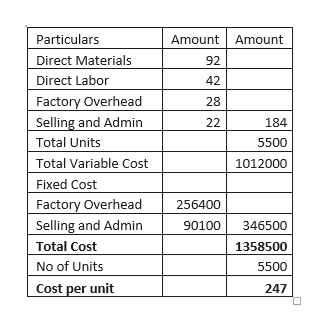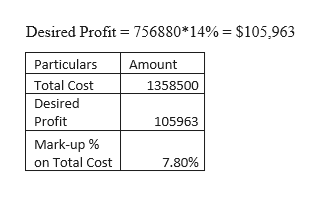# Smart Stream Inc. uses the total cost method of applying the cost-plus approach to product pricing. The costs of producing and selling 5,500 units of cell phones are as follows:Variable costs per unit: Fixed costs:     Direct materials\$ 92     Factory overhead\$256,400    Direct labor42     Selling and administrative expenses90,100    Factory overhead28       Selling and administrative expenses22            Total variable cost per unit\$184   Smart Stream desires a profit equal to a 14% return on invested assets of \$756,880.a.  Determine the total costs and the total cost amount per unit for the production and sale of 5,500 cellular phones. Round the cost per unit to two decimal places.Total cost\$Total cost amount per unit\$b.  Determine the total cost markup percentage for cellular phones. Round your answer to two decimal places. %c.  Determine the selling price of cellular phones. Round to the nearest cent.\$ per cellular phone

Question
16 views

Smart Stream Inc. uses the total cost method of applying the cost-plus approach to product pricing. The costs of producing and selling 5,500 units of cell phones are as follows:

 Variable costs per unit: Fixed costs: Direct materials \$ 92 Factory overhead \$256,400 Direct labor 42 Selling and administrative expenses 90,100 Factory overhead 28 Selling and administrative expenses 22 Total variable cost per unit \$184

Smart Stream desires a profit equal to a 14% return on invested assets of \$756,880.

a.  Determine the total costs and the total cost amount per unit for the production and sale of 5,500 cellular phones. Round the cost per unit to two decimal places.

 Total cost \$ Total cost amount per unit \$

b.  Determine the total cost markup percentage for cellular phones. Round your answer to two decimal places.
%

c.  Determine the selling price of cellular phones. Round to the nearest cent.
\$ per cellular phone

check_circle

Step 1

Cost plus approach involves addition of a mark-up value to the total cost.

Computation of Total Costhelp_outlineImage TranscriptioncloseAmount Amount Particulars Direct Materials 92 Direct Labor 42 Factory Overhead Selling and Admin 28 22 184 Total Units 5500 Total Variable Cost 1012000 Fixed Cost Factory Overhead 256400 Selling and Admin 90100 346500 Total Cost 1358500 No of Units 5500 Cost per unit 247 fullscreen
Step 2

Mark-up Va...help_outlineImage TranscriptioncloseDesired Profit = 756880*14% = \$105,963 Particulars Amount Total Cost 1358500 Desired Profit 105963 Mark-up % on Total Cost 7.80% fullscreen

### Want to see the full answer?

See Solution

#### Want to see this answer and more?

Solutions are written by subject experts who are available 24/7. Questions are typically answered within 1 hour.*

See Solution
*Response times may vary by subject and question.
Tagged in